Author Message
Martin_K

Joined: 09 Apr 2020
Posts: 227Posted: Sun Apr 11, 2021 1:46 pm    Post subject: some problems with 2 %pl optionsHello,

I added a new functionality to my plot to create height profile of the terrain from the surveyed points as follows: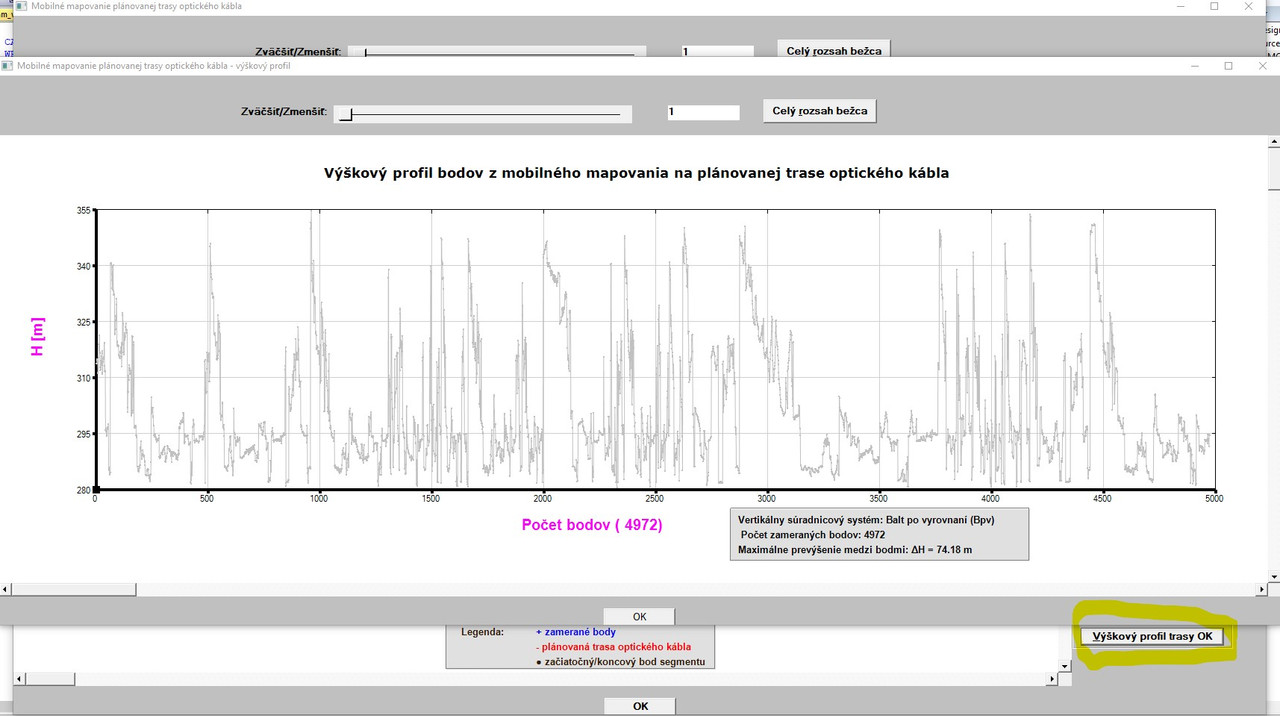Here, I encountered 2 problems:

1 - I wanted to hatch the area between bottom horizontal axis and the lines
which join the height points. I wanted to use for it the %PL option
LINK=COLUMNS (at least, I wanted to try it, how the graph will look).

Although compiling and linking was problem free, when invoking the
button for creating height profile, I get the following run-time error: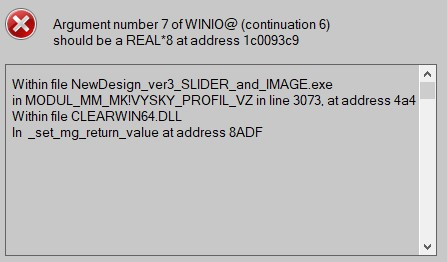the code in line 3073 where the error message points looks as follows:

 Code: iw=winio@('%`^pl&',gw+300,gh-200,L,REAL_L,H_MM,vyskovy_profil_VZ, pl_cb_vz)

So, it points to the argument No. 7, which is a name of a callback (at least I understand the message so). I do not understand, where is the problem (if I remove the option LINK=COLUMNS, everything runs OK).

2 - I wanted to use for the plot above the brown color. So I used (among others) also the %PL option COLOR=BROWN. Again, although compiling
and linking was fine, when invoking the CREATE HEIGHT PROFILE button, I get the following message: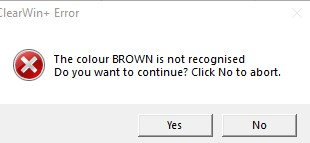When I click on YES, the plot is empty (only axes and their frames are drawn.

So, my question is - with the %PL option color, only BLUE,RED,YELLOW,GREEN,GREY and BLACK colors can be used (or what color could be used except the named above)?
Is there no possibility to use with the %PL option COLOR also RGB@(x,y,z)?

REMARK:
When I click on the full extents of slider it never returns fully from its current position to its initial position, although otherwise everything works fine. (I have to move the slider manually to its beginning)Kenneth_SmithJoined: 18 May 2012
Posts: 499
Location: Hamilton, Lanarkshire, Scotland.Posted: Sun Apr 11, 2021 3:18 pm    Post subject:Code: program brown_example use clrwin implicit none integer, parameter :: dp =kind(1.d0) integer, parameter :: n  = 10 real(kind=dp) :: x(1:n) = (/ (i,i=1,n) /), y(1:n) = (/ (i*i,i=1,n) /) integer iw iw = winio@('%mn[Exit]&','exit') call winop@('%pl[native,gridlines,frame,x_array]') call winop@('%pl[link=lines,symbol=6,colour=#964B00]')   !## The hexadecimal RGB code of Brown is #964B00. iw = winio@('%pl&',800,400,n,x,y) iw = winio@(' ') endMartin_K

Joined: 09 Apr 2020
Posts: 227Posted: Sun Apr 11, 2021 3:58 pm    Post subject:Thanks Ken - done! It works fine (I was unable to find in the docs that also hexadecimal numbers can be used with the %pl COLOUR option). Now, remains the problem with LINK=COLUMNS.Kenneth_SmithJoined: 18 May 2012
Posts: 499
Location: Hamilton, Lanarkshire, Scotland.Posted: Sun Apr 11, 2021 4:26 pm    Post subject:Martin,

Check the value of n_graphs in the earlier call to winop@.

The error message suggests that the call to %pl is not consistent with the
earlier winop specification.

Link = columns works in a simple example.

 Code: program brown_example2 use clrwin implicit none integer, parameter :: dp =kind(1.d0) integer, parameter :: n  = 150 real(kind=dp) :: x(1:n) = (/ (i,i=1,n) /), y(1:n) = (/ (i*i,i=1,n) /) integer iw integer, parameter :: pl_id = 1000 integer, external :: pl_cb iw = winio@('%mn[Exit]&','exit') call winop@('%pl[native,gridlines,frame,x_array,n_graphs=1]') call winop@('%pl[width=4,link=columns,symbol=6,colour=#964B00]')   !## The hexadecimal RGB code of Brown is #964B00. iw = winio@('%`^pl&',800,400,n,x,y,pl_id,pl_cb) iw = winio@(' ') end integer function pl_cb() pl_cb = 1 end function pl_cbMartin_K

Joined: 09 Apr 2020
Posts: 227Posted: Sun Apr 11, 2021 7:34 pm    Post subject:Ken,

After checking the input data, I discovered something which might have something to do with my problem
(with LINK=COLUMNS) and at the moment it is for me mystery.

Originally (when everything is OK and the plotting is fine - I mean the plot
with JPG image and the vector data in it as could be seen many times in my previous posts in other topics) I use for the plotting the code:

 Code: iw=winio@('%`^pl[full_mouse_input]&',gw,gh,N_PL,Y_OK_U,X_OK_U,handle_pl_ok,pl_cb)

where number of graphs/points represents the N_PL array which contains the values displayed here: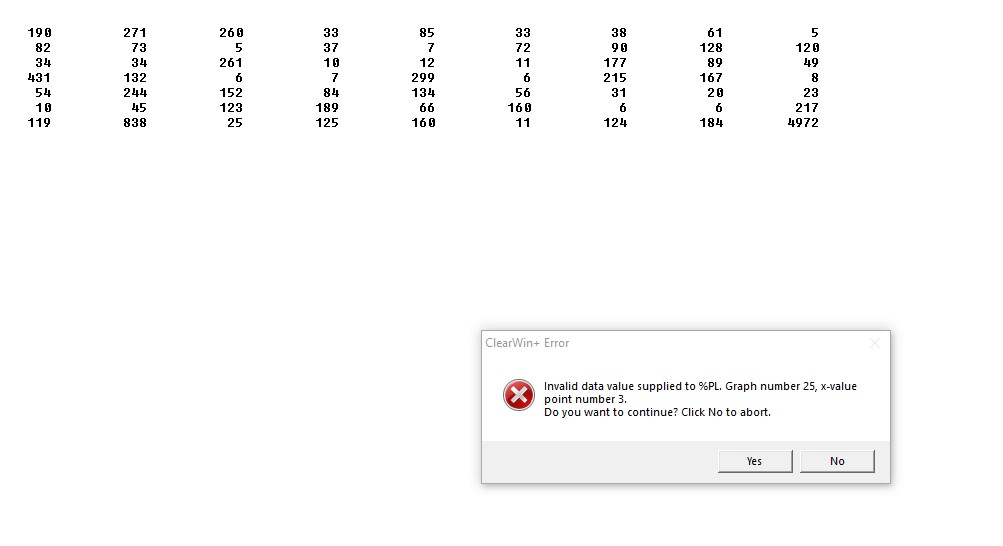So, in this particular case, the N_PL is an array (sum of points) of 62 graphs (total number of points = 6744 which are used for joining with lines) PLUS the 63rd graph
with 4972 points (no joining - surveyed points), hence total number of points is 11716. I think that this was done (with the N_PL arrays) because of STACKED option I used.

Then, based on your advise and to hunt after the run time error when using LINK=COLUMNS for the second and independent plot, I changed
the N_PL for the 1st plot to the scalar value of CBP which holds the total number of points 11716:

 Code: iw=winio@('%`^pl[full_mouse_input]&',gw,gh,CBP,Y_OK_U,X_OK_U,handle_pl_ok,pl_cb)

In this case, I get the error displayed above telling that in the Graph No. 25, x-value for point number 3 is invalid.

I inspected the input data for this part indicated by error message (in yellow):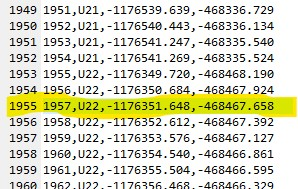Formally, there is no invalid data in the input file, otherwise my check
in the program would issue a warning and would stop the processing of data (please ignore that the numbering of points does not correspond
to the number of lines, the same is with feature coding U21, U22 which
should also indicate here the U25 belonging to the Graph 25, since in the Point ID´s and feature coding can be gaps in numbering and it has no influence on processing of the data.
I can number the points and use feature codes as I want, there is NO requirement for a continuous numbering/feature coding.

So, what happens here?

Be aware of the fact that the second and independent plot (height profile) draws ONLY 4972 points (surveyed points only).Martin_K

Joined: 09 Apr 2020
Posts: 227Posted: Mon Apr 12, 2021 11:13 am    Post subject:I thoroughly reviewed the situation with my previous post
and it has nothing to do with LINK=COLUMNS problem and is clear for me now.

So, now, I have a new situation. I found that in the 2nd plot (height profile)
I forgot to explicitly use the %PL options [native] and [n_graphs=1], since
I thought that both are default and there is no need to add them explicitly.

So, I explicitly added both above mentioned options to the 2nd plot
and removed the options [independent] which was there added by copying
some parts from my 1st %PL function.

Now, when I use LINK=COLUMNS for the 2nd plot, I get different run-time error message: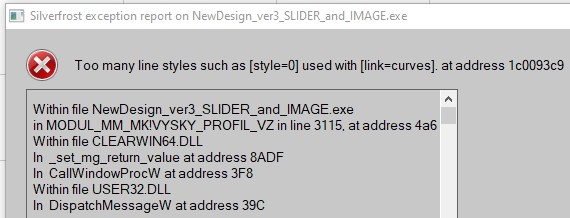I do not use too many line style and do not use the option LINK=CURVES.

Here is the whole code for my 2nd %PL function:

 Code: INTEGER FUNCTION VYSKY_PROFIL_VZ () IMPLICIT NONE IF (.NOT. ALLOCATED (REAL_L)) ALLOCATE (REAL_L(L)) DO i=1,L      REAL_L(i) = dble(i)    END DO write(pl_str,'("%pl[margin=(",I3,",",I3,",",I3,",",I3,")]")') mleft-50, mtop, mright-130, mbottom call winop@(pl_str) iw=winio@('%fn[Verdana]%ts[1.4]%bf%gf%sf&',hTitleFont) call winop_hdl@('%pl[title_hfont]&',hTitleFont)      CALL ENABLE_UTF8@(1) iw=winio@('%ww[no_border]%ca[Mobilné mapovanie plánovanej trasy optického kábla - výkový profil]%bg[grey]&')  iw=winio@('%2nl%5ta%bf Zväčiť/Zmeniť:%ta%50sl%ta%`bg[white]%`rf%sf&',zoom_vz,zoom_min_vz,zoom_max_vz,zoom_vz)  iw=winio@('%ta%bf%^bt[Celý &rozsah beca]%sf%2nl&',full_extents_cb_vz) CALL winop@('%pl[native]') CALL winop@('%pl[gridlines]') CALL winop@('%pl[x_sigfigs=8]') CALL winop@('%pl[y_sigfigs=6]') ! CALL winop@('%pl[link=columns]') CALL winop@('%pl[color=#964B00,link=lines,symbol=6,symbol_size=1]') CALL winop@('%pl[title="Výkový profil bodov z mobilného mapovania na plánovanej trase optického kábla"]') CALL winop@('%pl[x-axis=@,y-axis=@]') CALL winop@('%pl[x_array]') CALL winop@('%pl[frame,etched]') CALL winop@('%pl[axes_pen=4,smoothing=4,frame_pen=1]') CALL winop@('%pl[n_graphs=1]') iw=winio@("%vx&",vpage_step_vz,vmax_value_vz, vcur_val_vz) iw=winio@("%hx&",hpage_step_vz,hmax_value_vz, hcur_val_vz) iw=SELECT_GRAPHICS_OBJECT@(vyskovy_profil_VZ)        iw=winio@('%`^pl&',gw+300,gh-200,L,REAL_L,H_MM,vyskovy_profil_VZ, pl_cb_vz) iw=winio@('%dl&',0.5d0,timer_cb_vz) iw=winio@('%ff%nl%cn%tt[OK]&') iw=winio@('%ff%2nl&')                   iw=winio@(' ')  VYSKY_PROFIL_VZ = 1 END FUNCTION VYSKY_PROFIL_VZ

Is it important the sequence of the %PL options?
What´s wrong with the code above, when I get the run-time error
demonstrated above?Martin_K

Joined: 09 Apr 2020
Posts: 227Posted: Mon Apr 12, 2021 10:03 pm    Post subject:I finally catch the problem with the %PL [link=columns]:Here are my findings:

1. If you look in my code above, I have there (among others) the options

From my today´s experience I definitely can state the following:
The simultaneous presence of both options [link=columns] and [link=lines] in the same function does NOT work
at all, although compiling and linking is problem free. Therefore I got
the run-time error message declared in my previous post.

2. When I removed the option [link=lines], the run-time error was eliminated, however - it was not enough, since the plot was EMPTY, no points were drawn at all (only axes, frame and gridlines.
Look at my code above containing this line:

This code probably caused that the plot was empty. I found it by accident when I experimented with the sequence of %PL options to find the reason, why the plot is empty.

So, by guessing!!!, I changed the code in the above mentioned line as follows:

After this change, NEARLY everything works as presented in the picture above.

I am still hunting the following: When I removed the option LINK=LINES and changed the line above as described,
after invoking the button to draw height profile, I always get the following for the first time when plot is drawn:As can be seen here, INITIALLY the graph is shifted below horizontal axis.
At present, I do not know why. When I move (even slightly) with the vertical scrollbar, then plot is adjusted (and is in right position above
horizontal axis) as can be seen in the first picture in this post.

Here the question remains - why?

So, I would be thankful to anybody, if the above described behaviour can be confirmed generally or not. If yes, then
- it should be clearly stated in the docs that the options LINK=LINES and LINK=COLUMNS are in conflict and cannot be used at the same time
- and, when using option LINK=COLUMNS, there is a requirement for a

Thanks to anybody for trying to replicate my situation to see whether it is
a specific one or can be generally confirmed/excluded!

MartinKenneth_SmithJoined: 18 May 2012
Posts: 499
Location: Hamilton, Lanarkshire, Scotland.Posted: Thu Apr 15, 2021 10:50 am    Post subject:The code below replicates the reported problem and also demonstrates a simple fix.

 Code: module demo use clrwin implicit none integer, parameter :: dp = kind(1.d0), npoints=5 real(kind=dp) x(1:npoints), y(1:npoints) contains integer function gen_data() integer i, j j = -5   do i = 1, npoints     x(i) = j  ; y(i) = 1.d0/(j+10)     j = j+1   end do   gen_data = 2 end function gen_data integer function plot1() integer iw   call winop@('%pl[native,x_array,colour=blue,frame,gridlines,link=columns,width=8]')   iw = winio@('%pl&',800,800,npoints,x,y)   iw = winio@('%ff%cn%bt[OK]')   plot1 = 2 end function plot1 integer function plot2() integer iw character(len=250) plstr   call winop@('%pl[native,x_array,colour=blue,frame,gridlines,link=columns,width=8]')   write(plstr,"('%pl[y_min=',F6.4,']')" ) minval(y) !  print*, plstr   call winop@(plstr)   iw = winio@('%pl&',800,800,npoints,x,y)   iw = winio@('%ff%cn%bt[OK]')   plot2 = 2 end function plot2 end module demo program main use demo i = gen_data() i = plot1() i = plot2() end program mainMartin_K

Joined: 09 Apr 2020
Posts: 227Posted: Thu Apr 15, 2021 12:25 pm    Post subject:Many thanks Ken, great simple fix! Done and it works fine! Nevertheless, I would also like to formally hear the answers (if possible) whether: 1 - the %PL options LINK=LINES and LINK=COLUMNS are in conflict when they are used simultaneously (so, their simultaneous use is not allowed) 2 - when using the option LINK=COLUMNS, after its use is there a need for specific sequence of additional %PL options which must be maintained and may not be altered. I think such info would be very useful for everybody. One more question: I would also need to have in axis titles/captions (sometimes) also a subscript/superscript (%sd,%su) in the text. I tried to use %sd with DRAW_CHARACTERS@, where previously I tried to write it in an output string, but unsuccessfully. Whereas in standard winio@ operations it works problem free, I would like to see a simple example (if possible) how to achieve it for using with draw_characters@. Thanks in advance!Kenneth_SmithJoined: 18 May 2012
Posts: 499
Location: Hamilton, Lanarkshire, Scotland.Posted: Fri Apr 16, 2021 11:35 am    Post subject:I suspect that it would be impossible to describe every possible combination of parameters/options that users may try to program in the documentation. Here are my own observations as a user - I can only guess what is "under the bonnet" of the Clearwin.dll:

[link=columns] and [link=lines] cannot be used when [n_graphs=1]. It should be obvious that this will create a run time error.

When using [link=columns] a width value must be specified [width=2] say, columns (or bars) are not displayed if this width value is not specified.

When using [link=columns] it is not possible to also draw a symbol at the data point (effectively symbol=0). (Unlikely to be a requirement in practice.)

Remembering that the link/symbol/colour instructions are processed sequentially i.e. first link instruction is applied to graph 1, second to graph 2 and so on. The same applies to colour and symbol.

This can result in some issues with the non-appearance of symbols when mixing multiple graphs with [link=lines] and [link=columns]. This can be avoided by always assigning symbol=0 to the graph which has [link=columns].

The [width] value is mandatory when using [link=columns] and when specified applies to all graphs (including those using [link=lines]).

The observations above can be demonstrated by making modifications to the function plot3 in the following code. As it stands, the code demonstrates that it is possible to combine [link=lines] and [link=columns] for n_graphs=2.
 Code: module demo use clrwin implicit none integer, parameter :: dp = kind(1.d0), npoints=50 real(kind=dp) x(1:npoints), y(1:npoints), z(1:npoints) contains integer function gen_data() integer i, j j = 1   do i = 1, npoints     x(i) = j  ; y(i) = 1.d0/(j+10)     j = j+1   end do   z = 2.5d0*y   gen_data = 2 end function gen_data integer function plot3() integer iw integer n(1:2)   n = npoints   call winop@('%pl[native,n_graphs=2,x_array,frame,gridlines, width=2]')   call winop@('%pl[link=columns,symbol=0,  colour=red]')        !# Remove symbol=0 here and no symbols are shown!   call winop@('%pl[link=lines,  symbol=6,  colour=blue]')   iw = winio@('%pl&',800,800,n,x,y,z)   iw = winio@('%ff%cn%bt[OK]')   plot3 = 2 end function plot3 end module demo program main use demo i = gen_data() i = plot3() end program main

For displaying captions with mathematical equations I create an external graphics file containing the equation and simply import the file to the appropriate location.Martin_K

Joined: 09 Apr 2020
Posts: 227Posted: Fri Apr 16, 2021 9:14 pm    Post subject:Many thanks for sharing of your your valuable experiences with %PL options Ken! This will avoid (at least for me) to waste my time when using %PL and its options! It is a pity that such important info is not included in the documentation. MartinPaulLaidlerJoined: 21 Feb 2005
Posts: 7068
Location: Salford, UKPosted: Sat Apr 17, 2021 7:43 am    Post subject:Martin It is only a matter of resources. Those who provide this service for you also need time to sleep.Martin_K

Joined: 09 Apr 2020
Posts: 227Posted: Mon Apr 19, 2021 11:59 am    Post subject:Thanks Paul! Of course, everybody - except God - has his limits. With every of my post my aim is - except my own learning as a novice - to point to some possible/potential problems of the product indicated by some procedures I used. This can be used by the authors of the product to improve their own product and make steady advance and refining. Any other interpretations of my posts is totally incorrect. Best wishes! MartinDisplay posts from previous: All Posts1 Day7 Days2 Weeks1 Month3 Months6 Months1 Year Oldest FirstNewest First
 All times are GMT + 1 Hour Page 1 of 1